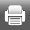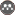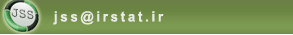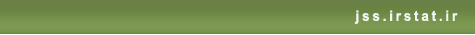[Home ] [Archive]   [ فارسی ]Volume 4, Issue 1 (9-2010)J. of Stat. Sci. 2010, 4(1): 89-103 Back to browse issues page
D-efficiency of D-optimal Designs for Poisson Model with Random Intercept
Abstract:   (19279 Views)
The main part of optimal designs in the mixed effects models concentrates on linear models and binary models. Recently, Poisson models with random effects have been considered by some researchers. In this paper, an especial case of the mixed effects Poisson model, namely Poisson regression with random intercept is considered. Experimental design variations are obtained in terms of the random effect variance and indicated that the variations depend on the variance parameter. Using D-efficiency criterion, the impression of random effect on the experimental setting points is studied. These points are compared with the optimal experimental setting points in the corresponding model without random effect. We indicate that the D-efficiency depends on the variance of random effect.
Keywords: D-optimality, Quasi-Likelihood, D-efficiency, Poisson Regression Model, Quasi-information Matrix, Random Effect .
Type of Study: Applied | Subject: Applied Statistics
Send email to the article author